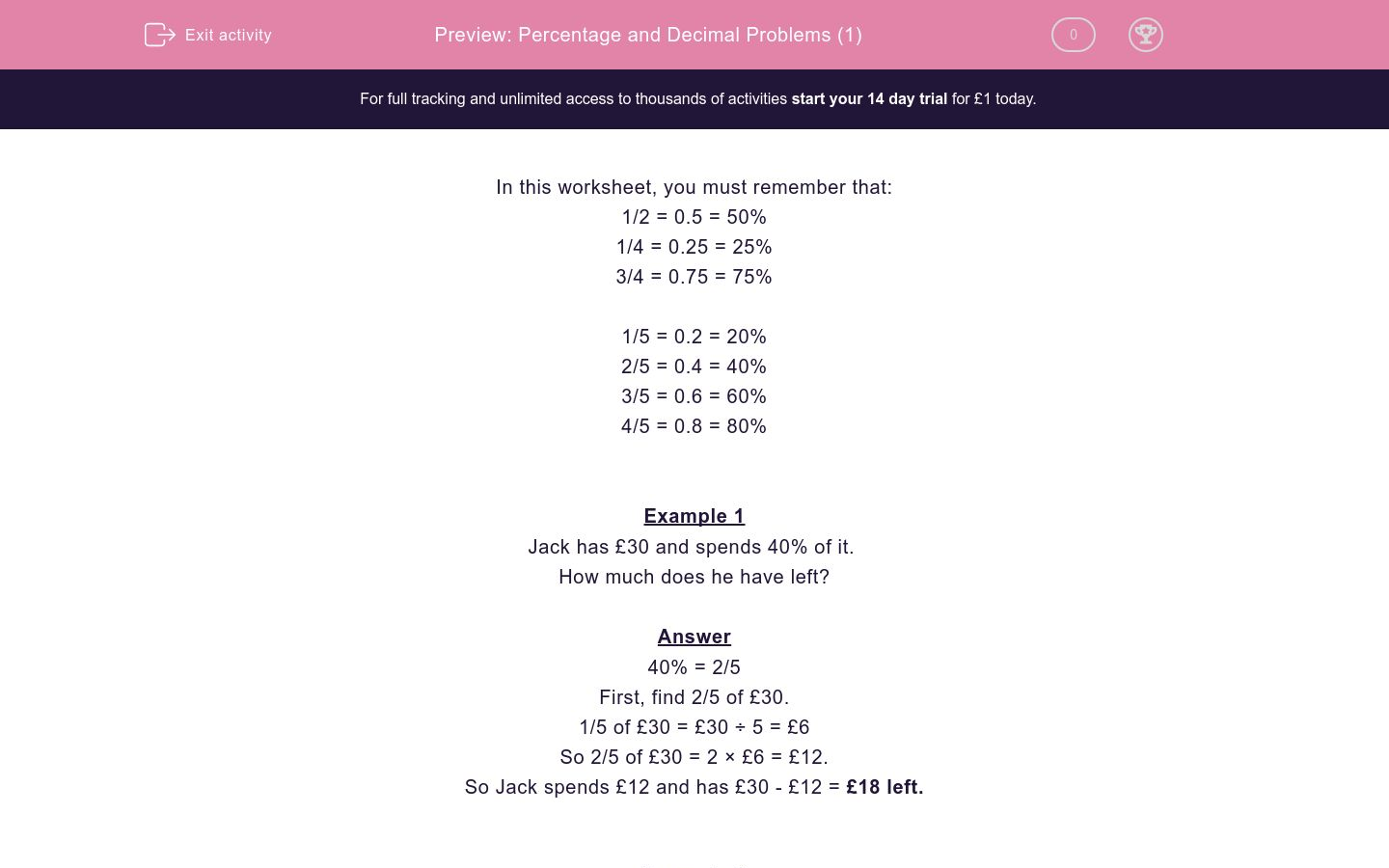# Percentage and Decimal Problems (1)

In this worksheet, students solve problems which require knowing the equivalents of a half, quarters and fifths.Key stage:  KS 2

Curriculum topic:   Maths and Numerical Reasoning

Curriculum subtopic:   Percentages

Difficulty level:#### Worksheet Overview

In this worksheet, you must remember that:

1/2 = 0.5 = 50%

1/4 = 0.25 = 25%

3/4 = 0.75 = 75%

1/5 = 0.2 = 20%

2/5 = 0.4 = 40%

3/5 = 0.6 = 60%

4/5 = 0.8 = 80%

Example 1

Jack has £30 and spends 40% of it.

How much does he have left?

40% = 2/5

First, find 2/5 of £30.

1/5 of £30 = £30 ÷ 5 = £6

So 2/5 of £30 = 2 × £6 = £12.

So Jack spends £12 and has £30 - £12 = £18 left.

Example 2

Which is bigger, 3/4 of £100 or 80% of £90?

1/4 of £100 = £100 ÷ 4 = £25

3/4 of £100 = 3 × £25 = £75

80% = 4/5

1/5 of £90 = £90 ÷ 5 = £18

4/5 of £90 = 4× £18 = £72

£75 is bigger than £72.

So 3/4 of £100 is bigger than 80% of £90.

### What is EdPlace?

We're your National Curriculum aligned online education content provider helping each child succeed in English, maths and science from year 1 to GCSE. With an EdPlace account you’ll be able to track and measure progress, helping each child achieve their best. We build confidence and attainment by personalising each child’s learning at a level that suits them.

Get started